# Bayes' theorem

##  Bayesian Inference

Bayes' theorem is about conditional probabilities. Probability is about sets of outcomes. We start by assuming that these outcomes are equally likely. Suppose we have a bag full of balls, each ball is either red or blue. Each ball is also either Small or Big. Taking a ball from the bag is an outcome.

Red Blue Total
Small
20
40
60
Big
10
30
40
Total
30
70
100

The conditional probability of a ball taken from the bag being Red if we already know it is Big is 10/40. This is written,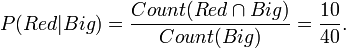$P(Red|Big) = \frac{Count(Red \cap Big)}{Count(Big)} = \frac{10}{40}.$$P(Big|Red) = \frac{Count(Big \cap Red)}{Count(Red)} = \frac{10}{30}.$

These are conditional probabilities. P(Red | Big) means,

 First I found that the ball was Big.  What then is the probability of it being red.


The probabilities for a a ball being red P(Red) is,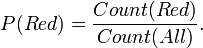$P(Red) = \frac{Count(Red)}{Count(All)}.$

Note that P(Red | Big) has no meaning by itself. Instead probability has two sets,

• The set of events that register success.
• The domain from which those events are taken.

Note that,

P(Red) = P(Red | All).

The probabilities for a a ball being Big P(Big) is,$P(Big) = \frac{Count(Big)}{Count(All)}.$

Now the probability of a ball being Red and Big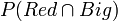$P(Red \cap Big)$ is,$P(Red\cap Big) = \frac{Count(Red\cap Big)}{Count(All)}.$

or,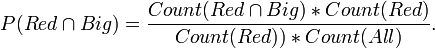$P(Red\cap Big) = \frac{Count(Red\cap Big) * Count(Red)}{Count(Red)) * Count(All)}.$

so,$P(Red\cap Big) = P(Big|Red) * P(Red).$

similarly,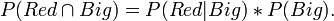$P(Red\cap Big) = P(Red|Big) * P(Big).$

so the result is,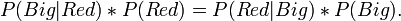$P(Big|Red) * P(Red) = P(Red|Big) * P(Big). \!$

This is Bayes' theorm usually written as,$P(Big|Red) = \frac{P(Red | Big)\, P(Big)}{P(Red)}.$

it is also true that,$p(Red) = P(Red|Big) * P(Big) + P(Red|Small) * P(Small). \!$

so,$P(Big|Red) = \frac{P(Red | Big)\, P(Big)}{P(Red|Big) * P(Big) + P(Red|Small) * P(Small)}.$